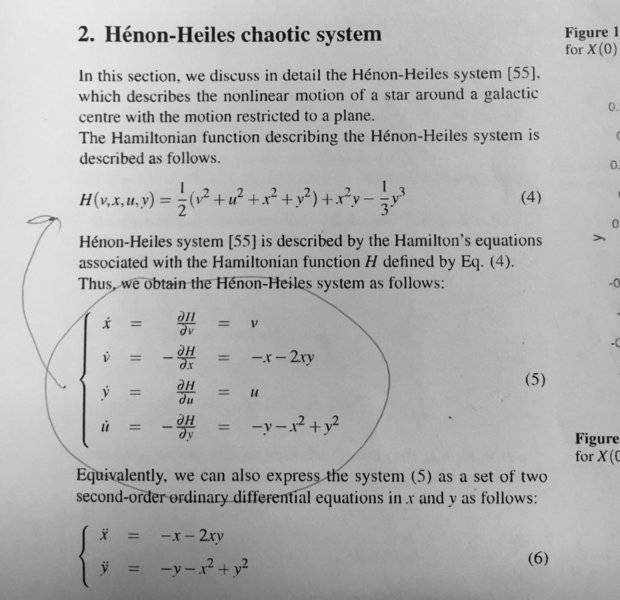# How to simplify this Equation

• I
• ohaited

#### ohaited

Hey, I have this chaotic system. It is a modified Hamiltonian Chaotic System and it is based on Henon-Heiles chaotic system. So I have this functions (as shown below). I want to know how can I make it as a discrete function. Like, how can I know the value for x dot and y dot.1. Prefer to know it in a simple way, take it as someone who doesn't have physics fundamental but want to know about these two chaotic system. Really appreciate you help.

Oh, and yeah. If there any misinterpret for keyword. Do correct me :)

Thanks :)

#### Attachments

Prefer to know it in a simple way
Isn't equation (5) in your picture the simplest representation ? You do have four variables to integrate, not two ...

Isn't equation (5) in your picture the simplest representation ? You do have four variables to integrate, not two ...

Oh really? I don't quite get what you want to tell me. Can you tell me a little bit more detail? Like how do I integrate all these variables?

P/s: I'm new with all these chaotic system, this study is for the purpose of studying chaotic map for my crypto-system.

Your initial variables are ##x,\dot x, y, \dot y## and all four develop in time according to the equations (5)

Your initial variables are ##x,\dot x, y, \dot y## and all four develop in time according to the equations (5)
Oh, okay. So it is a time dependent variables. So how do I integrate those variables. The ##\dot x, \dot y## as far as I knew it is differential equation. How to make it difference equation.

##x_{n+1} = x_{n} + v_n \Delta t ##
##v_{n+1} = -x_{n} -2 x_n y_n\Delta t \ ## etc.

This is the simples approach, called Euler integration. It won't give you nice stable orbits, so you may way to search for better integration methods.